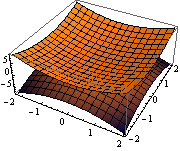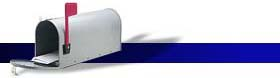####Syllabus: Computer Algebra Systems (Math 119)

Course Description: This one hour, one credit course has been designed as an introduction to computer algebra, or symbolic algebra systems, using the application Mathematica. The focus is immersive learning engagement in using computational calculation and graphic tools in scientific and technical problem solving.

Learning Objectives: Upon successful completion of this course, the student should be able to use Mathematica as a mathematical computing package to support their numerical and symbolic computing efforts in the physical sciences, engineering and social sciences. The emphasis will be on how to gain facility in using it as a mathematical and scientific calculator, scripting engine, and as an end-user computer progarmming language (EUP).

1. Familiarization and Overview via Review of Intermediate Algebra

• Tool package: Workspace Access & Use, Control and Management
• Concepts: Numbers, Arithmetic; Basic Geometry, Algebra, Trigonometry
2. Calculations with Manipulations of Data Types, Structures and Collections
• Numbers, Symbolizations, Variables, Expressions, Formulas, Equations
• Lists, Vectors, Matrices, Tables, sigma-pi reductions, Computational Statistics
• Functions and Functional Programming, Recursion, Algebraic Calculus
• Graphics, Plots, Presentation and Visualization Graphics
3. End User "Programming" (EUP) and Scripting for Science and Technology Calculations
• Procedural and Imperative, Program Control Structures, Patterns
• Recursive Programming, Divide and Conquer, Greedy Algorithms
• Rule-based Programming & Table-driven programming
• Graphics Programming, Animation
4. Advanced Topics (if time permits)
• Front Ends, Interfacing to the Package, Integration with other CAS (e.g. Excel)
• 3-D Graphics, Simulations
• Packages, "Modular" Programming
• Matrix Computation, Analytics, Numerical Analysis

Textbook (required)
Don, Eugene; Mathematica (Second Edition); McGraw Hill; ISBN: 978-0-07-160828-2

Grading Policy: All students are expected to attend all lectures. Students are expected to do most of the programming exercises outside of the lecture hours. There will be four, equally weighted exams. In addition, projects and homework will be periodically assigned.